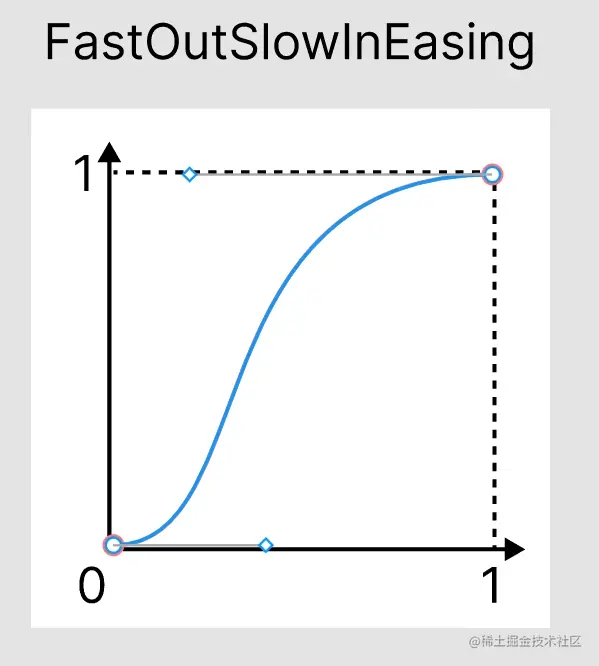﻿ Android动效Compose贝塞尔曲线动画规格详解_Android_脚本之家
Android# Android动效Compose贝塞尔曲线动画规格详解

## 正文

```val borderRadius by animateIntAsState(
targetValue = if (isRound) 100 else 0,
animationSpec = tween(
durationMillis = 3000,
easing = LinearEasing
)
)
```x轴可以理解为时间进度，而y轴可以理解动画的进度，可以看出该图为线性曲线，即从头到尾保持一样的速度。关于各个不同的曲线产生不同的动画效果可以看下Android官网的Easing API，里面有比较多的动图来演示。

```@Stable
fun <T> tween(
durationMillis: Int = DefaultDurationMillis,
delayMillis: Int = 0,
easing: Easing = FastOutSlowInEasing
): TweenSpec<T> = TweenSpec(durationMillis, delayMillis, easing)
```

```val FastOutSlowInEasing: Easing = CubicBezierEasing(0.4f, 0.0f, 0.2f, 1.0f)
val LinearOutSlowInEasing: Easing = CubicBezierEasing(0.0f, 0.0f, 0.2f, 1.0f)
val FastOutLinearInEasing: Easing = CubicBezierEasing(0.4f, 0.0f, 1.0f, 1.0f)
val LinearEasing: Easing = Easing { fraction -> fraction }
```

## 贝塞尔曲线• 第一个参数为起始点把手的x坐标
• 第二个参数为起始点把手的y坐标
• 第三个参数为终点把手的x坐标
• 第四个参数为终点把手的y坐标
```CubicBezierEasing(0.4f, 0.0f, 0.2f, 1.0f)
```## 解析动画曲线## 曲线源码分析

```@Stable
fun interface Easing {
fun transform(fraction: Float): Float
}
```

`CubicBezierEasing`则继承了`Easing`，并实现了transform方法

```@Immutable
class CubicBezierEasing(
private val a: Float,
private val b: Float,
private val c: Float,
private val d: Float
) : Easing {
...
private fun evaluateCubic(a: Float, b: Float, m: Float): Float {
return 3 * a * (1 - m) * (1 - m) * m +
3 * b * (1 - m) * /*    */ m * m +
/*                      */ m * m * m
}
override fun transform(fraction: Float): Float {
if (fraction > 0f && fraction < 1f) {
var start = 0.0f
var end = 1.0f
while (true) {
val midpoint = (start + end) / 2
val estimate = evaluateCubic(a, c, midpoint)
if ((fraction - estimate).absoluteValue < CubicErrorBound)
return evaluateCubic(b, d, midpoint)
if (estimate < fraction)
start = midpoint
else
end = midpoint
}
} else {
return fraction
}
}
...
}
```